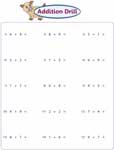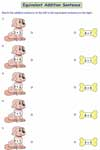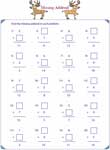It contains printable standard addition worksheets with two word problems at the end of each worksheet.Grab 'em All

Grab 'em All

Practice makes perfect. Use these column addition drills pdf worksheets for kindergarten and 1st grade to practice single-digit addition.15 per page:

25 per page:

50 per page:

75 per page:

100 per page:

In this section, the addends are arranged horizontally in each problem.15 per page:

25 per page:

50 per page:

75 per page:

100 per page:

These simple addition worksheets include single-digit numbers arranged in a both vertical and horizontal form.20 per page:

30 per page:

50 per page:

100 per page:

Add the numbers in these printable worksheets to find the sum. Color the picture based on the color guide. Identify the mystery picture.Column:

Horizontal:

Mixed:

Circle the fruits on each tree that have the sum shown on the trunk.Matching Equivalent Sums

Match the single-digit addition sentence on the left to the equivalent addition sentence on the right. Each pdf worksheet has unique theme.You have one of the addends and the sum. Find the missing addend that makes the sum.Find out the number that makes the addition equation true in each problem. Caterpillar themes are used.Cut-Glue Activity

Compare the addition sentence and glue the correct picture on the box. Unique theme is used in each printable worksheet.Easy:

Moderate:

Add the single-digit numbers on the petals and write the answer at the center of each flower of these pdf worksheets for grade 1.